Thermodynamics and Propulsion

17.4 Muddiest Points on Chapter 17

MP 17..1   How do we know that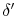is not a fluid property?

The term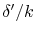represents the resistance to heat transfer for a unit area. The resistance to heat transfer per unit area (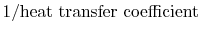) can be computed for cases of laminar flow, or measured experimentally where we cannot compute it, and it is found that for the same state variables, it can have a range of values of several orders of magnitude depending on the parameters I described (Reynolds number, surface condition, surface shape ...). Put another way, if the value of the resistance is affected by the surface condition (smooth, bumpy, corrugated, etc.) how can the resistance be just a property of the fluid?

MP 17..2   What is the analogy'' that we are discussing? Is it that the equations are similar?

While the equations are similar, the concepts are deeper than that. The analogy is drawn between the heat transfer process (transfer of heat represented by heat flux) and the momentum transfer process (transfer of momentum represented by shear stress).

MP 17..3   In what situations does the Reynolds analogy not work?''

The Reynolds Analogy is just that. It is not a law of nature, but rather a plausible hypothesis that allows useful estimation of the heat transfer coefficients in many situations in which little or no explicit heat transfer information exists. In the form we have derived it, the Reynolds Analogy is appropriate for use in air, but it is not strictly applicable if there are pressure gradients, or if the Prandtl number (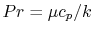) is not unity. However, the conceptual framework provided by the analogy has been found useful enough that the analogy has been extended (in a more complex form, as briefly discussed in class) for application to these situations.

MP 17..4   In the expression, what is?is the area normal to the heat flow. For a turbine blade, for example, it would be the outer surface area of the blade.

MP 17..5   It seems that we have simplified convection a lot. Is finding the heat transfer coefficient, h, really difficult?

We have indeed simplified convection a lot.'' We will look at heat transfer by convection in more depth in a few lectures, but to answer the question in a few sentences, finding the heat transfer coefficient is a difficult problem, because it necessitates determining the fluid dynamics; the latter is key to predictions of heat transfer. Even with present computational power, calculating the flow around aerospace devices with the accuracy needed to be confident about heat transfer coefficients is not by any means a standard'' calculation. For some circumstances, it is still beyond the state of the art. We will concentrate on describing (i) the basic mechanisms of convective heat transfer and (ii) ways of estimating the heat transfer coefficient from known fluid dynamic information.

MP 17..6   What does the K'' in the contact resistance formula stand for?

The definition of the resistance comes out of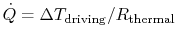. The units of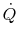are Watts/meter2, so the units of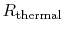are [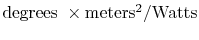]. The K'' is thus the symbol for Kelvin, or degree centigrade.

MP 17..7   In the equation for the temperature in a cylinder  (17.25), what is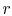''?

The variabledenotes the radial coordinate, in other words the location of the point at which we want to know the temperature.

UnifiedTP